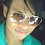-->

# Control Flow and Logic Functions in Matlab

Most of the computer programming provides a flow control feature, as well as MATLAB. One example of Control flow in MATLAB is:
1. Looping for
2. Looping while
3. If-else statement

### LOOPING FOR

The basic syntax for looping for is

for i = array
block statment
end

every time you pass this loop, the value of the looping variable i is the next value in the array. Colon notation is usually used to generate a sequence of numbers. Example:

for i = 1: 10

commands between for and end are executed once in each array. Some looping may be nested, where each loop ends with end.

### LOOPING WHILE

Looping while will execute an uncertain number of times. in general the syntax of the loop is

while expression
block statement
end

commands between while and end will be executed as long as the expression is correct. Note that in MATLAB a non-zero scalar is declared true. Usually a scalar is used in expressions, but an array can also be used. If the expression is an array, then all the elements in the array must be correct to execute the commands.

### IF-ELSE STATEMENT

sometimes, commands must be executed based on certain conditions. An if-else statement is suitable for that case. In general the syntax of the statement is

if expression
block statement
elseif expression
block statement
else
block statement
end

only one end is needed to end the if, elseif, and else statements. Commands will be executed if the expression is correct.

### RELATION AND LOGIC OPERATORS IN MATLAB

In practice while looping and if-else statements often use relations operators and logical operators. The relation operator is used to test whether two values ​​are the same, or one value is greater, or smaller than the other value. Whereas Logic Operators are used to combine logic statements to produce other logical values.

 Operator Definition Type < less than Relation <= less than or equal to Relation > more than Relation >= More than or equal to Relation == Together with Relation ~= Not equal to Relation & And Logic | Or Logic ~ Not or not Logic

By applying a relation operator, the result is a logical value (true / false / 1) or (false / false / 0).

The following is a simple example of using a relation operator,

While the example of using a logical operator is

### SOME EXAMPLES OF MATLAB FLOW AND LOGIC CONTROL

In this section several examples of simple programs in the form of m-files will be given to illustrate the use of theories discussed earlier.

Use Looping For (save with filename example4.m)

function sum=example4(x)
n=length(x);
sum=0;
for k=1:n
sum=sum+x(k);
end

Use of Looping While (save with file name example5.m)

function example5(x)
n=length(x);
m=median(x);
xsort=sort(x);
i=1;
while xsort(i)<=m
i=i+1;
end
disp ('Number of data <= median exists'); disp (i-1);
disp ('Amount of data> median exists'); disp (n-i + 1);
end

Use the IF-ELSE Statement (save with the file name example6.m)

function example6(x)
if x>0
disp ('x value is positive');
elseif x <0
disp ('x value is negative');
else
disp ('x value is 0');
end

### 1 Response to "Control Flow and Logic Functions in Matlab"

1.